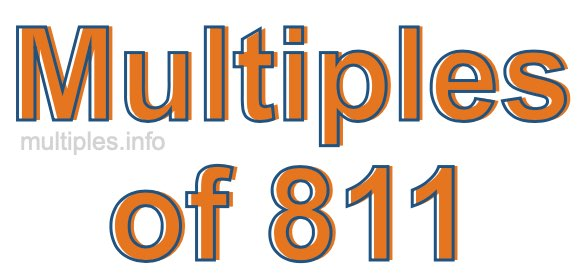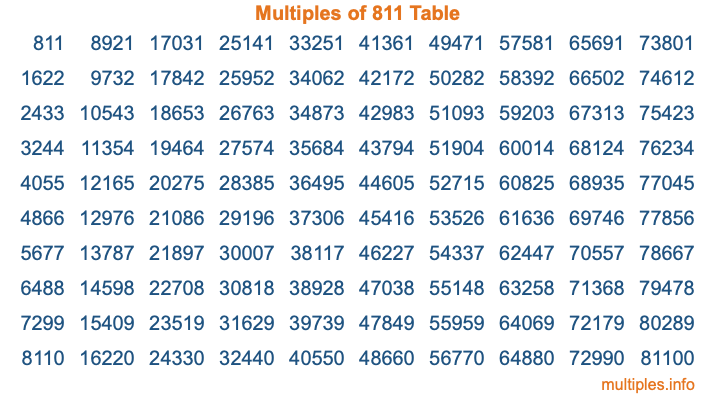Multiples of 811Welcome to the Multiples of 811 page. Here we will first teach you everything you will ever need to know about the multiples of 811, and then give you a study guide summary of everything we taught you to make sure you remember it all. Use this page to look up facts and learn information about the multiples of 811. This page will make you a multiples of eight hundred eleven expert!

Definition of Multiples of 811
Multiples of 811 are all the numbers that when divided by 811 equal an integer. Each of the multiples of 811 are called a multiple. A multiple of 811 is created by multiplying 811 by an integer.

Therefore, to create a list of multiples of 811, you start with 1 multiplied by 811, then 2 multiplied by 811, then 3 multiplied by 811, and so on for as long as you want. Thus, the list of the first five multiples of 811 is 811, 1622, 2433, 3244, and 4055. To see a larger list of multiples of 811, see the printable image of Multiples of 811 further down on this page. We also have a category where you can choose any nth multiple of 811.

Multiples of 811 Checker
The Multiples of 811 Checker below checks to see if any number of your choice is a multiple of 811. In other words, it checks to see if there is any number (integer) that when multiplied by 811 will equal your number. To do that, we divide your number by 811. If the the quotient is an integer, then your number is a multiple of 811.

Is  a multiple of 811?

Least Common Multiple of 811 and ...
A Least Common Multiple (LCM) is the lowest multiple that two or more numbers have in common. This is also called the smallest common multiple or lowest common multiple and is useful to know when you are adding our subtracting fractions. Enter one or more numbers below (811 is already entered) to find the LCM.

Check out our LCM Calculator if you need more details about the Least Common Multiple or if you need the LCM for different numbers for adding and subtraction fractions.

nth Multiple of 811
As we stated above, 811 is the first multiple of 811, 1622 is the second multiple of 811, 2433 is the third multiple of 811, and so on. Enter a number below to find the nth multiple of 811.

th multiple of 811

Multiples of 811 vs Factors of 811
811 is a multiple of 811 and a factor of 811, but that is where the similarities end. All postive multiples of 811 are 811 or greater than 811. All positive factors of 811 are 811 or less than 811.

Below is the beginning list of multiples of 811 and the factors of 811 so you can compare:

Multiples of 811: 811, 1622, 2433, 3244, 4055, etc.

Factors of 811: 1, 811

As you can see, the multiples of 811 are all the numbers that you can divide by 811 to get a whole number. The factors of 811, on the other hand, are all the whole numbers that you can multiply by another whole number to get 811.

It's also interesting to note that if a number (x) is a factor of 811, then 811 will also be a multiple of that number (x).

Multiples of 811 vs Divisors of 811
The divisors of 811 are all the integers that 811 can be divided by evenly. Below is a list of the divisors of 811.

Divisors of 811: 1, 811

The interesting thing to note here is that if you take any multiple of 811 and divide it by a divisor of 811, you will see that the quotient is an integer.

Multiples of 811 Table
Below is an image of the first 100 multiples of 811 in a table. The table is in chronological order, column by column. The first column has the first ten multiples of 811, the second column has the next ten multiples of 811, and so on.The Multiples of 811 Table is also referred to as the 811 Times Table or Times Table of 811. You are welcome to print out our table for your studies.

Negative Multiples of 811
Although not often discussed or needed in math, it is worth mentioning that you can make a list of negative multiples of 811 by multiplying 811 by -1, then by -2, then by -3, and so on, to get the following list of negative multiples of 811:

-811, -1622, -2433, -3244, -4055, etc.

Multiples of 811 Summary
Below is a summary of important Multiples of 811 facts that we have discussed on this page. To retain the knowledge on this page, we recommend that you read through the summary and explain to yourself or a study partner why they hold true.

There are an infinite number of multiples of 811.

A multiple of 811 divided by 811 will equal a whole number.

811 divided by a factor of 811 equals a divisor of 811.

The nth multiple of 811 is n times 811.

The largest factor of 811 is equal to the first positive multiple of 811.

811 is a multiple of every factor of 811.

811 is a multiple of 811.

A multiple of 811 divided by a divisor of 811 equals an integer.

811 divided by a divisor of 811 equals a factor of 811.

Any integer times 811 will equal a multiple of 811.

Multiples of a Number
Here you can get the multiples of another number, all with the same attention to detail as we did for multiples of 811 on this page.

Multiples of
Multiples of 812
Did you find our page about multiples of eight hundred eleven educational? Do you want more knowledge? Check out the multiples of the next number on our list!